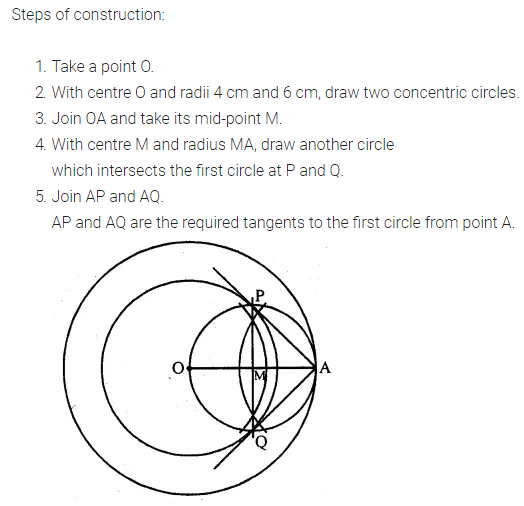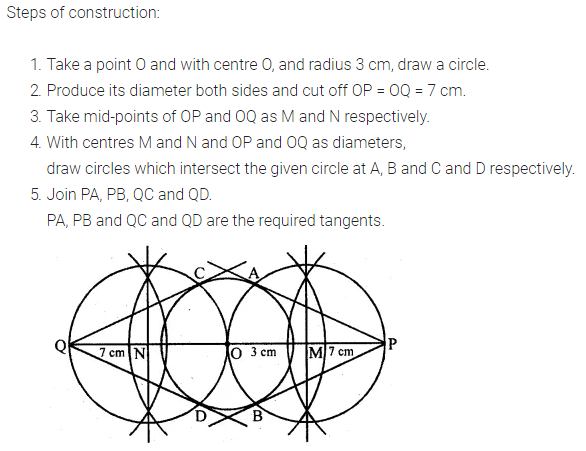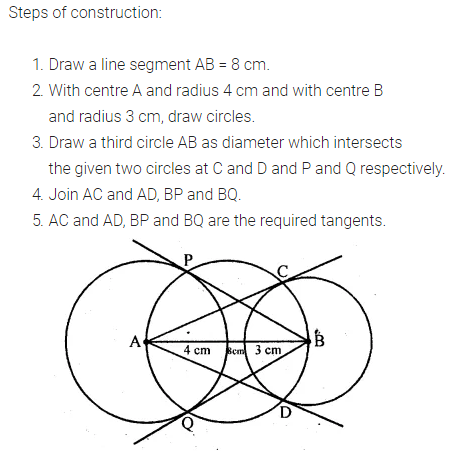# ML Aggarwal Class 10 Solutions for ICSE Maths Chapter 16 Constructions Ex 16.1

## ML Aggarwal Class 10 Solutions for ICSE Maths Chapter 16 Constructions Ex 16.1

ML Aggarwal Class 10 Solutions for ICSE Maths Chapter 16 Constructions Ex 16.1

Question 1.
Use a ruler and compass only in this question.
(i) Draw a circle, centre O and radius 4 cm.
(ii) Mark a point P such that OP = 7 cm.
Construct the two tangents to the circle from P. Measure and record the length of one of the tangents.
Solution:Question 2.
Draw a line AB = 6 cm. Construct a circle with AB as diameter. Mark a point P at a distance of 5 cm from the mid-point of AB. Construct two tangents from P to the circle with AB as diameter. Measure the length of each tangent
Solution:Question 3.
Construct a tangent to a circle of radius 4cm from a point on the concentric circle of radius 6 cm and measure its length. Also, verify the measurement by actual calculation.
Solution:Question 4.
Draw a circle of radius 3 cm. Take two points P and Q on one of its extended diameter each at a distance of 7 cm from its centre. Draw tangents to the circle from these two points P and Q.
Solution:Question 5.
Draw a line segment AB of length 8 cm. Taking A as centre, draw a circle of radius 4 cm and taking B as centre, draw another circle of radius 3 cm. Construct tangents to each circle from the centre of the other circle.
Solution: